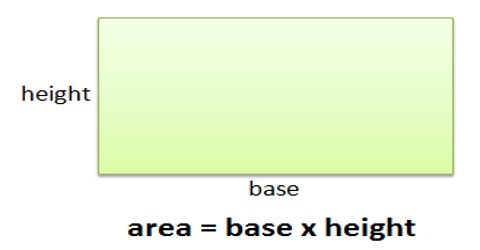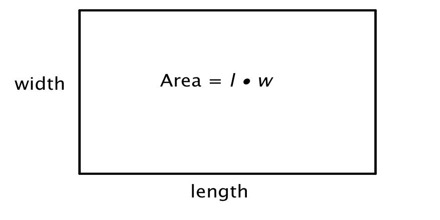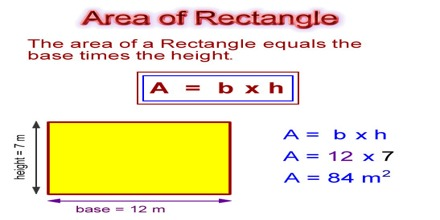Mathematic

# How to find the Area of a Rectangle?Calculating the Area of a Rectangle

A rectangle is a four-sided plane figure, or any quadrilateral, with four right angles. Opposite sides of a rectangle are parallel and equal in length. The area of a rectangle can be found by multiplying the base times the height. So, it is the number of square units it takes to completely fill a rectangle. Formula: Width × Height/Length.where,

• w- is the width
• l- is the length / height

So, to calculate the area of a rectangle, multiply the length of the rectangle by its width. You need to know the length and width of the rectangle. If a rectangle has a base of length 6 meter and a height of 4 inches, its area is 12 × 7 = 84 square meter.Example: The size of something can be measured in a lot of different ways. For example, the size that fits you best is typically how tall you are. Or, the size of a swimming pool might be how deep it is.